ybarrap

•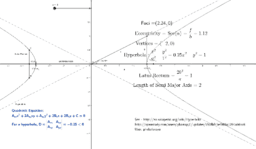Hyperbola

Activity

ybarrap

•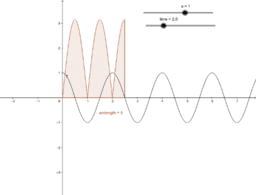A particle moves according to the equation x=acosPi t the di

Activity

ybarrap

•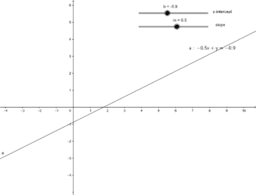Find the slope and the y-intercept of the equation

Activity

ybarrap

•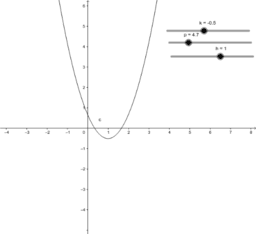Describe the location of the vertex

Activity

ybarrap

•Which function has the greatest starting value?

Activity

ybarrap

•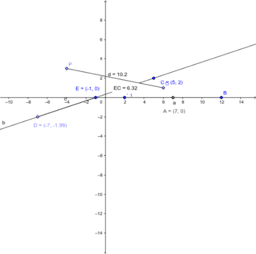Midpoints

Activity

ybarrap

•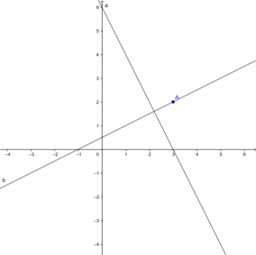write an equation in slope intercept form for a line contain

Activity

ybarrap

•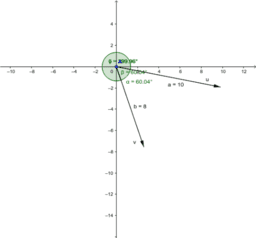If |u| = 10, |v| = 8, and the angle formed between them is .

Activity

ybarrap

•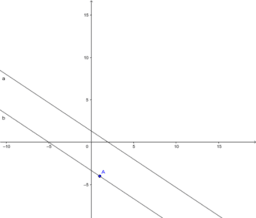Write the standard form of the line that is parallel to pt

Activity

ybarrap

•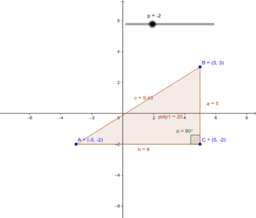The vertices of a right triangle

Activity

ybarrap

•blacksmith1

Activity

ybarrap

•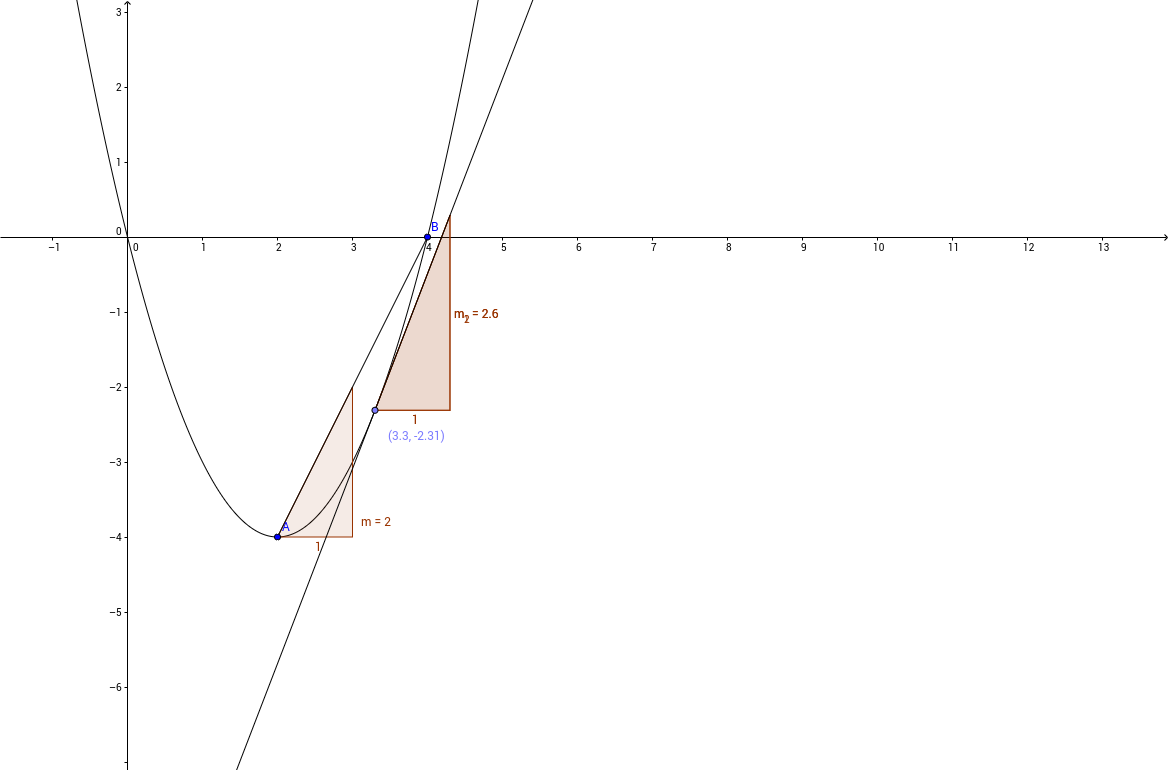MVT example

Activity

ybarrap

•Activity

ybarrap